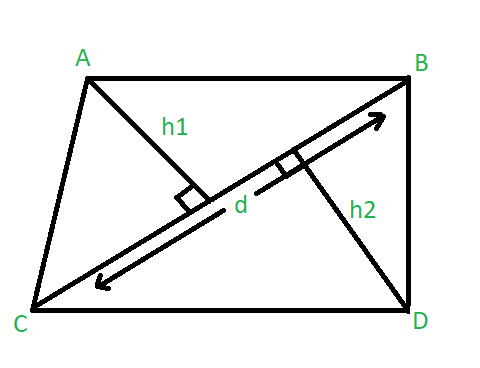# Find the area of quadrilateral when diagonal and the perpendiculars to it from opposite vertices are given

Given three integers d, h1, h2 where d represents the length of the diagonal of a quadrilateral. h1 and h2 represents the lengths of the perpendiculars to the given diagonal from the opposite vertices. The task is to find the area of the Quadrilateral.Examples:

Input : d= 6, h1 = 4, h2 = 3
Output : 21

Input : d= 10, h1 = 8, h2 = 10
Output : 90

## Recommended: Please try your approach on {IDE} first, before moving on to the solution.

Approach :
Area of the quadrilateral is the sum of the areas of both triangles. We know that the area of the triangle is 1/2*base*height.

Therefore, the area of a quadrilateral can be calculated as :

Area = 1/2 * d * h1 + 1/2 * d * h2
= 1/2 * d * ( h1 + h2 )

Below is the implementation of the above approach :

## C++

 `// C++ program to find the area of quadrilateral ` `#include ` `using` `namespace` `std; ` ` `  `// Function to find the area of quadrilateral ` `float` `Area(``int` `d, ``int` `h1, ``int` `h2) ` `{ ` `    ``float` `area; ` ` `  `    ``area = 0.5 * d * (h1 + h2); ` ` `  `    ``return` `area; ` `} ` ` `  `// Driver code ` `int` `main() ` `{ ` `    ``int` `d = 6, h1 = 4, h2 = 3; ` ` `  `    ``cout << ``"Area of Quadrilateral = "` `<< (Area(d, h1, h2)); ` ` `  `    ``return` `0; ` `} `

## Java

 `// Java program to find the area of quadrilateral ` `class` `GFG  ` `{ ` ` `  `    ``// Function to find the area of quadrilateral ` `    ``static` `float` `Area(``int` `d, ``int` `h1, ``int` `h2)  ` `    ``{ ` `        ``float` `area; ` ` `  `        ``area = (``float``) ``0.5` `* d * (h1 + h2); ` ` `  `        ``return` `area; ` `    ``} ` ` `  `    ``// Driver code ` `    ``public` `static` `void` `main(String[] args)  ` `    ``{ ` `        ``int` `d = ``6``, h1 = ``4``, h2 = ``3``; ` ` `  `        ``System.out.println(``"Area of Quadrilateral = "` `+  ` `                                      ``Area(d, h1, h2)); ` `    ``} ` `} ` ` `  `// This code is contributed by Princi Singh `

## Python3

 `# Python3 program to find ` `# the area of quadrilateral ` ` `  `# Function to find the  ` `# area of quadrilateral ` `def` `Area(d, h1, h2): ` ` `  `    ``area ``=` `0.5` `*` `d ``*` `(h1 ``+` `h2); ` ` `  `    ``return` `area; ` ` `  `# Driver code ` `if` `__name__ ``=``=` `'__main__'``: ` `     `  `    ``d ``=` `6``;  ` `    ``h1 ``=` `4``;  ` `    ``h2 ``=` `3``; ` ` `  `    ``print``(``"Area of Quadrilateral = "``,  ` `                  ``(Area(d, h1, h2))); ` ` `  `# This code is contributed by Rajput-Ji `

## C#

 `// C# program to find the area of quadrilateral ` `using` `System; ` ` `  `class` `GFG  ` `{ ` `     `  `// Function to find the area of quadrilateral ` `static` `float` `Area(``int` `d, ``int` `h1, ``int` `h2) ` `{ ` `    ``float` `area; ` ` `  `    ``area = (``float``)0.5 * d * (h1 + h2); ` ` `  `    ``return` `area; ` `} ` ` `  ` `  `// Driver code ` `public` `static` `void` `Main() ` `{ ` `    ``int` `d = 6, h1 = 4, h2 = 3; ` `     `  `    ``Console.WriteLine(``"Area of Quadrilateral = "` `+  ` `                                 ``Area(d, h1, h2));  ` `} ` `} ` ` `  `// This code is contributed by nidhiva `

Output:

```Area of Quadrilateral = 21
```

My Personal Notes arrow_drop_upCheck out this Author's contributed articles.

If you like GeeksforGeeks and would like to contribute, you can also write an article using contribute.geeksforgeeks.org or mail your article to contribute@geeksforgeeks.org. See your article appearing on the GeeksforGeeks main page and help other Geeks.

Please Improve this article if you find anything incorrect by clicking on the "Improve Article" button below.

Article Tags :
Practice Tags :

Be the First to upvote.

Please write to us at contribute@geeksforgeeks.org to report any issue with the above content.